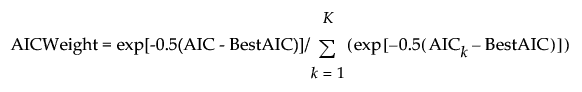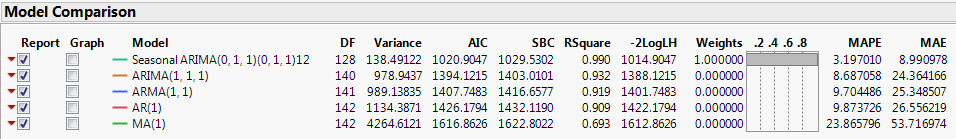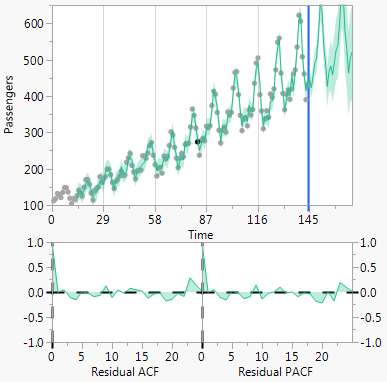Publication date: 05/24/2021

## Model Comparison Report

Once a model is fit, the Model Comparison Report is displayed in the report window. This report contains the Model Comparison table and plots for the models. Each time a new model is fit, a new row is added to the Model Comparison table, with a unique color-coding. The Model Comparison table summarizes the fit statistics for each model and is used to compare several models fitted to the same time series. By default, the models are sorted by the AIC statistic, in increasing order. To sort the model by a different statistic, right-click any column in the Model Comparison Table and select Sort by Column. Alternatively, you can also click on the column header to sort. For definitions of the fit statistics, see Model Summary Table. The only fit statistic that is unique to the Model Comparison Table is Weights. This fit statistic is the normalized AIC Weight. The AIC Weight for a model is calculated as follows:K is the total number of models, AICk is the AIC value for model k, and BestAIC is the AIC value for the model with the minimum AIC value.

If you specify a holdback set, the fit statistics that are computed on the training data are not shown in the Model Comparison table. Instead, the following metrics to determine the forecasting performance of the individual models on the holdback set are computed: Root Mean Square Error (RMSE), Mean Square Error (MSE), Mean Absolute Percentage Error (MAPE), and Mean Absolute Error (MAE). By default, the models are sorted by the RMSE statistic, in increasing order. To sort the model by a different statistic, right-click any column in the Model Comparison Table and select Sort by Column. Alternatively, you can also click on the column header to sort. For definitions of the fit statistics, see Model Summary Table.

Caution: If you do not specify a holdback set and fit a combination of state space smoothing models and ARIMA models, a caution message is shown at the top of the Model Comparison table. In this case, the state space smoothing models are not comparable to the ARIMA models because likelihood values and likelihood-based information criteria, such as the AIC statistic, are not comparable between the two different model classes. However, if you specify a holdback set, the models can be compared because the RMSE statistic is used.

Figure 18.12 Model Comparison TableYou can select which full model reports are shown in the report window using the Report check box. Two model plots appear to the right of the Model Comparison table. The top plot is a time series plot of the data, forecasts, and prediction limits. Below that are plots of the autocorrelation and partial autocorrelation functions. You can select which models are displayed on the model plots using the Graph check box.

Figure 18.13 Model Plots### Model Comparison Report Options

Each model in the Model Comparison report has the following red triangle menu options:

Fit New

Opens a specification window for the model. You can change the settings to fit a different model.

Simulate Once

(Not available if a holdback set is specified.) Provides one simulation of the model to the upper end of the time axis. The simulation is shown on the Model Comparison time series plot.

Simulate More

(Not available if a holdback set is specified.) Provides the specified number of simulations of the model to the upper end of the time axis. The simulations are shown on the Model Comparison time series plot.

Remove Model Simulation

(Not available if a holdback set is specified.) Removes the simulations for the model.

Remove All Simulation

(Not available if a holdback set is specified.) Removes the simulations for all models.

Generate Simulation

(Not available if a holdback set is specified.) Generates simulations for the model, and stores the results in a data table. You can specify the random seed, number of simulations, and the number of forecast periods.

Set Seed

(Not available if a holdback set is specified.) Specifies the seed for generating the simulated trajectories.

Remove Fit

Removes the corresponding model from the report window.

Remove All Models

Removes all models that are listed in the Model Comparison table from the report window.

Remove Unselected

Removes all models that do not have the Report check box selected from the report window.

Remove Selected

Removes all models that have the Report check box selected from the report window.

Hide All Reports

Hides all of the models that are listed in the Model Comparison table from the report window. This is equivalent to deselecting all of the Report check boxes.

Hide All Graphs

Hides all of the models that are listed in the Model Comparison table from the Model Plots. This is equivalent to deselecting all of the Graph check boxes.

Note: The Time Series platform treats excluded rows as missing values. If the last several observations in the data table are excluded or missing, the simulation options in the Model Comparison Report will not work properly. The simulation procedure uses the last several observations of the time series. If the last several observations are missing, the simulation produces all missing values into the future.